Tanishq Gautam — October 16, 2020

## Introduction

Have you ever stumbled upon a dataset or an image and wondered if you could create a system capable of differentiating or identifying the image?

The concept of image classification will help us with that. Image Classification is one of the hottest applications of computer vision and a must-know concept for anyone wanting to land a role in this field.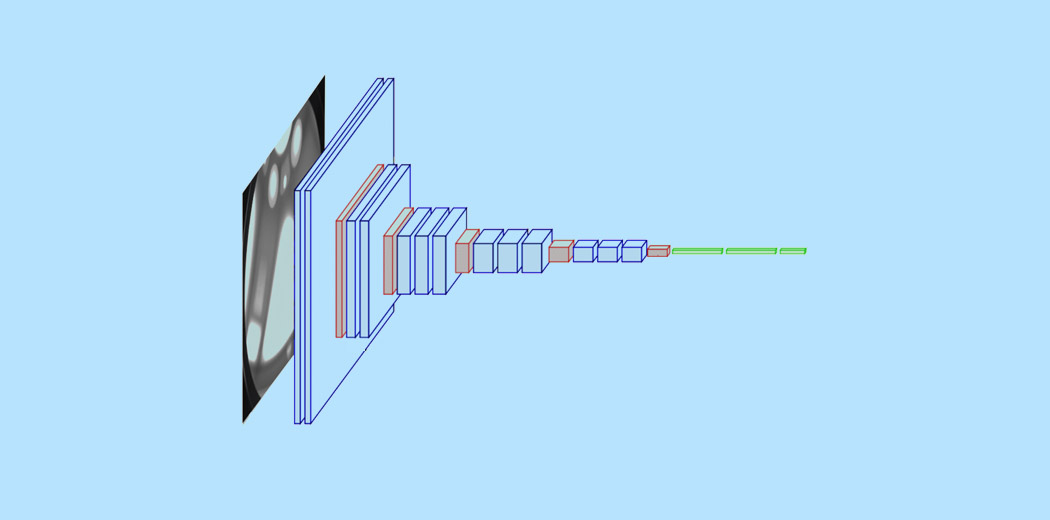In this article, we will see a very simple but highly used application that is Image Classification. Not only will we see how to make a simple and efficient model to classify the data but also learn how to implement a pre-trained model and compare the performance of the two.

By the end of the article, you will be able to find a dataset of your own and implement image classification with ease.

Prerequisites before you get started:

Sound interesting? So get ready to create your very own Image Classifier!

1. Image Classification
2. Understanding the Problem Statement
3. Setting up the Image Data
4. Let’s Build our Image Classification Model
1. Data Preprocessing
2. Data Augmentation
3. Model definition and training
4. Evaluating Results
5. The Art of Transfer Learning
1. Import base MobileNetV2 model
2. Fine-tuning
3. Training
4. Evaluating results
6. What’s Next?

## What is Image Classification?

Image Classification is the task of assigning an input image, one label from a fixed set of categories. This is one of the core problems in Computer Vision that, despite its simplicity, has a large variety of practical applications.

Let’s take an example to better understand. When we perform image classification our system will receive an image as input, for example, a Cat. Now the system will be aware of a set of categories and its goal is to assign a category to the image.

This problem might seem simple or easy but it is a very hard problem for the computer to solve. As you might know, the computer sees a grid of numbers and not the image of a cat as how we see it. Images are 3-dimensional arrays of integers from 0 to 255, of size Width x Height x 3. The 3 represents the three color channels Red, Green, Blue.

So how can our system learn to identify this image? By using Convolutional Neural Networks. Convolutional neural networks or CNN’s are a class of deep learning neural networks that are a  huge breakthrough in image recognition. You might have a basic understanding of CNN’s by now, and we know CNN’s consist of convolutional layers, Relu layers, Pooling layers, and Fully connected dense layers.

To read about Image Classification and CNN’s in detail you can check out the following resources:-

• https://www.analyticsvidhya.com/blog/2020/02/learn-image-classification-cnn-convolutional-neural-networks-3-datasets/
• https://www.analyticsvidhya.com/blog/2019/01/build-image-classification-model-10-minutes/

Now that we have an understanding of the concepts, let’s dive into how an image classification model can be built and how it can be implemented.

## Understanding the Problem Statement

Consider the following image: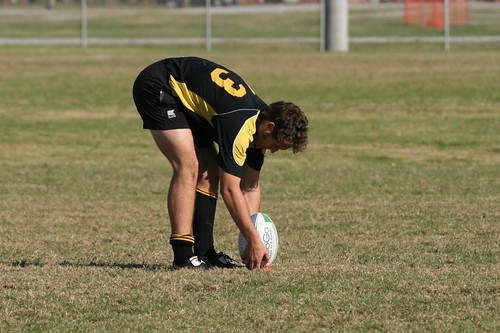A person well versed with sports will be able to recognize the image as Rugby. There could be different aspects of the image that helped you identify it as Rugby, it could be the shape of the ball or the outfit of the player. But did you notice that this image could very well be identified as a Soccer image?

Let’s consider another image:-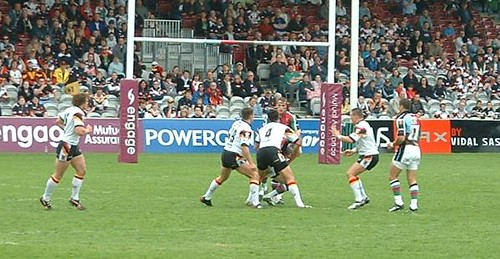What do you think this image represents? Hard to guess right? The image to the untrained human eye can easily be misclassified as soccer, but in reality, is a rugby image as we can see the goal post behind is not a net and bigger in size. The question now is can we make a system that can possibly classify the image correctly.

That is the idea behind our project here, we want to build a system that is capable of identifying the sport represented in that image. The two classification classes here are Rugby and Soccer. The problem statement can be a little tricky since the sports have a lot of common aspects, nonetheless, we will learn how to tackle the problem and create a good performing system.

## Setting up Our Image Data

Since we are working on an image classification problem I have made use of two of the biggest sources of image data, i.e, ImageNet, and Google OpenImages. I implemented two python scripts that we’re able to download the images easily. A total of 3058 images were downloaded, which was divided into train and test. I performed an 80-20 split with the train folder having 2448 images and the test folder has 610. Both the classes Rugby and Soccer have 1224 images each.

Our data structure is as follows:-

• Input – 3058
• Train – 2048
• Rugby – 1224
• Soccer – 1224
• Test – 610
• Rugby – 310
• Soccer – 310

## Let’s Build our Image Classification Model!

#### Step 1:- Import the required libraries

Here we will be making use of the Keras library for creating our model and training it. We also use Matplotlib and Seaborn for visualizing our dataset to gain a better understanding of the images we are going to be handling. Another important library to handle image data is Opencv.

```import matplotlib.pyplot as plt
import seaborn as sns

import keras
from keras.models import Sequential
from keras.layers import Dense, Conv2D , MaxPool2D , Flatten , Dropout
from keras.preprocessing.image import ImageDataGenerator

from sklearn.metrics import classification_report,confusion_matrix

import tensorflow as tf

import cv2
import os

import numpy as np```

Next, let’s define the path to our data. Let’s define a function called get_data() that makes it easier for us to create our train and validation dataset. We define the two labels ‘Rugby’ and ‘Soccer’ that we will use. We use the Opencv imread function to read the images in the RGB format and resize the images to our desired width and height in this case both being 224.

```labels = ['rugby', 'soccer']
img_size = 224
def get_data(data_dir):
data = []
for label in labels:
path = os.path.join(data_dir, label)
class_num = labels.index(label)
for img in os.listdir(path):
try:
img_arr = cv2.imread(os.path.join(path, img))[...,::-1] #convert BGR to RGB format
resized_arr = cv2.resize(img_arr, (img_size, img_size)) # Reshaping images to preferred size
data.append([resized_arr, class_num])
except Exception as e:
print(e)
return np.array(data)```
```Now we can easily fetch our train and validation data.
train = get_data('../input/traintestsports/Main/train')
val = get_data('../input/traintestsports/Main/test')```

#### Step 3:- Visualize the data

Let’s visualize our data and see what exactly we are working with. We use seaborn to plot the number of images in both the classes and you can see what the output looks like.

```l = []
for i in train:
if(i == 0):
l.append("rugby")
else
l.append("soccer")
sns.set_style('darkgrid')
sns.countplot(l)```

Output: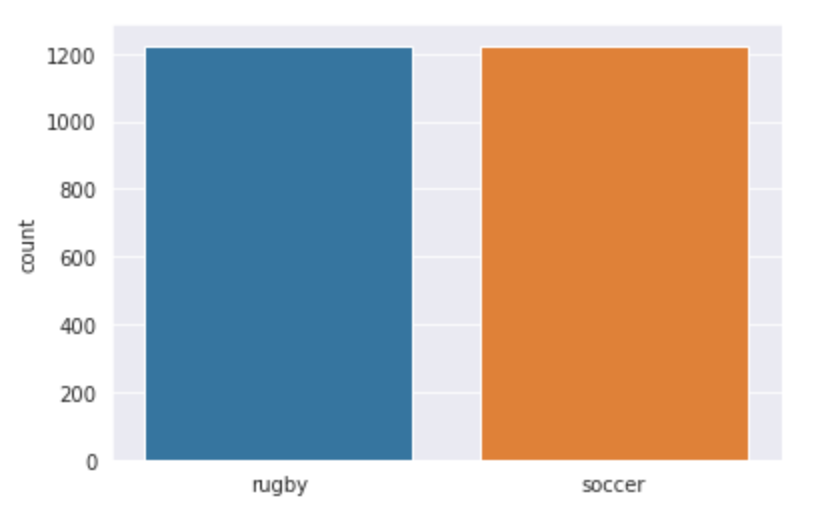Let us also visualize a random image from the Rugby and Soccer classes:-

```plt.figure(figsize = (5,5))
plt.imshow(train)
plt.title(labels[train])```

Output:-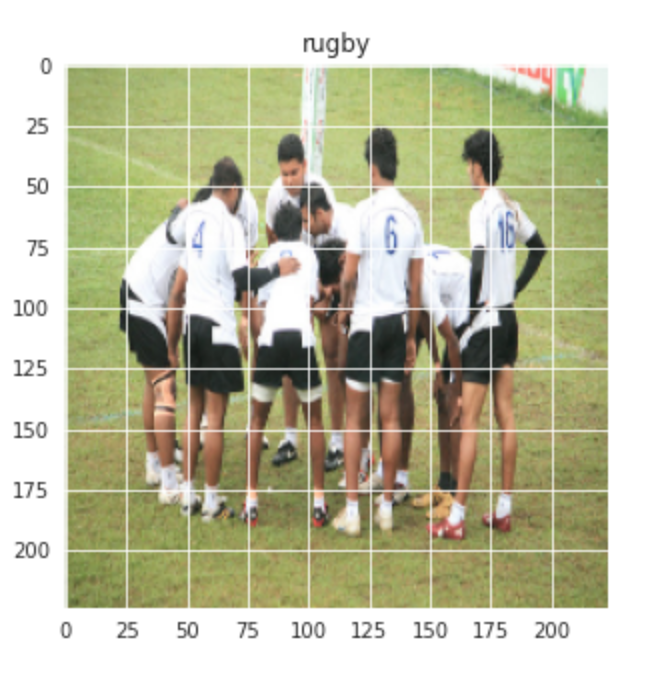Similarly for Soccer image:-

```plt.figure(figsize = (5,5))
plt.imshow(train[-1])
plt.title(labels[train[-1]])```

Output:-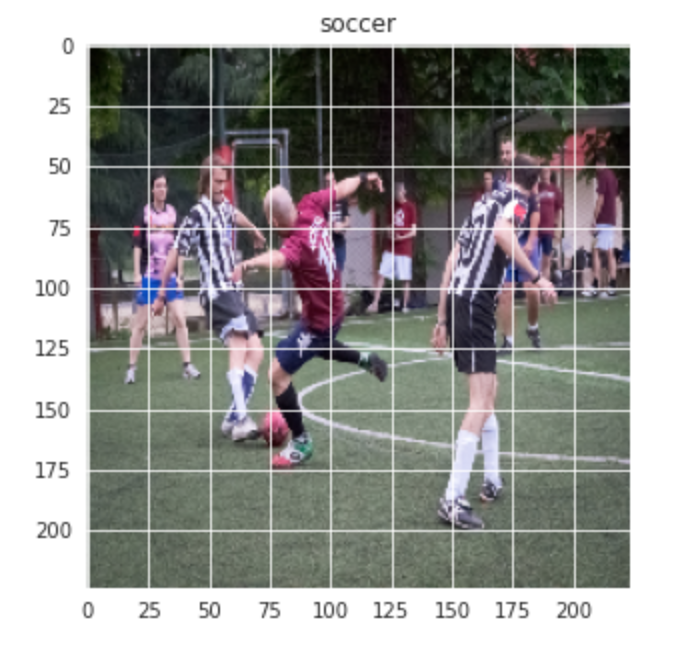#### Step 4:- Data Preprocessing and Data Augmentation

Next, we perform some Data Preprocessing and Data Augmentation before we can proceed with building the model.

```x_train = []
y_train = []
x_val = []
y_val = []

for feature, label in train:
x_train.append(feature)
y_train.append(label)

for feature, label in val:
x_val.append(feature)
y_val.append(label)

# Normalize the data
x_train = np.array(x_train) / 255
x_val = np.array(x_val) / 255

x_train.reshape(-1, img_size, img_size, 1)
y_train = np.array(y_train)

x_val.reshape(-1, img_size, img_size, 1)
y_val = np.array(y_val)```

Data augmentation on the train data:-

```datagen = ImageDataGenerator(
featurewise_center=False,  # set input mean to 0 over the dataset
samplewise_center=False,  # set each sample mean to 0
featurewise_std_normalization=False,  # divide inputs by std of the dataset
samplewise_std_normalization=False,  # divide each input by its std
zca_whitening=False,  # apply ZCA whitening
rotation_range = 30,  # randomly rotate images in the range (degrees, 0 to 180)
zoom_range = 0.2, # Randomly zoom image
width_shift_range=0.1,  # randomly shift images horizontally (fraction of total width)
height_shift_range=0.1,  # randomly shift images vertically (fraction of total height)
horizontal_flip = True,  # randomly flip images
vertical_flip=False)  # randomly flip images

datagen.fit(x_train)```

Step 5:- Define the Model

Let’s define a simple CNN model with 3 Convolutional layers followed by max-pooling layers. A dropout layer is added after the 3rd maxpool operation to avoid overfitting.

```model = Sequential()

model.summary()```

Let’s compile the model now using Adam as our optimizer and SparseCategoricalCrossentropy as the loss function. We are using a lower learning rate of 0.000001 for a smoother curve.

```opt = Adam(lr=0.000001)
model.compile(optimizer = opt , loss = tf.keras.losses.SparseCategoricalCrossentropy(from_logits=True) , metrics = ['accuracy'])```

Now, let’s train our model for 500 epochs since our learning rate is very small.

`history = model.fit(x_train,y_train,epochs = 500 , validation_data = (x_val, y_val))`

#### Step 6:- Evaluating the result

We will plot our training and validation accuracy along with training and validation loss.

```acc = history.history['accuracy']
val_acc = history.history['val_accuracy']
loss = history.history['loss']
val_loss = history.history['val_loss']

epochs_range = range(500)

plt.figure(figsize=(15, 15))
plt.subplot(2, 2, 1)
plt.plot(epochs_range, acc, label='Training Accuracy')
plt.plot(epochs_range, val_acc, label='Validation Accuracy')
plt.legend(loc='lower right')
plt.title('Training and Validation Accuracy')

plt.subplot(2, 2, 2)
plt.plot(epochs_range, loss, label='Training Loss')
plt.plot(epochs_range, val_loss, label='Validation Loss')
plt.legend(loc='upper right')
plt.title('Training and Validation Loss')
plt.show()```

Let’s see what the curve looks like:-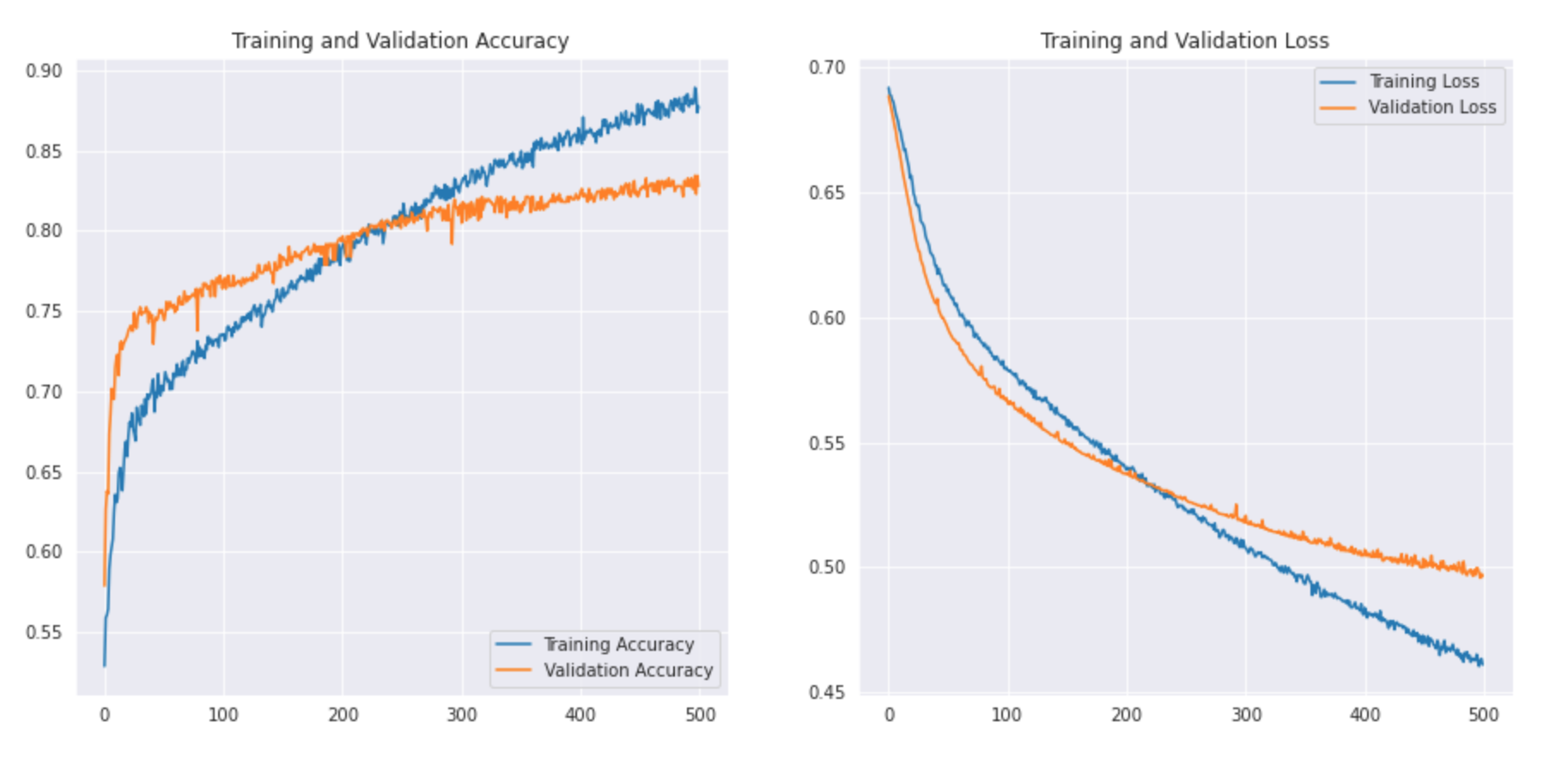We can print out the classification report to see the precision and accuracy.

```predictions = model.predict_classes(x_val)
predictions = predictions.reshape(1,-1)
print(classification_report(y_val, predictions, target_names = ['Rugby (Class 0)','Soccer (Class 1)']))```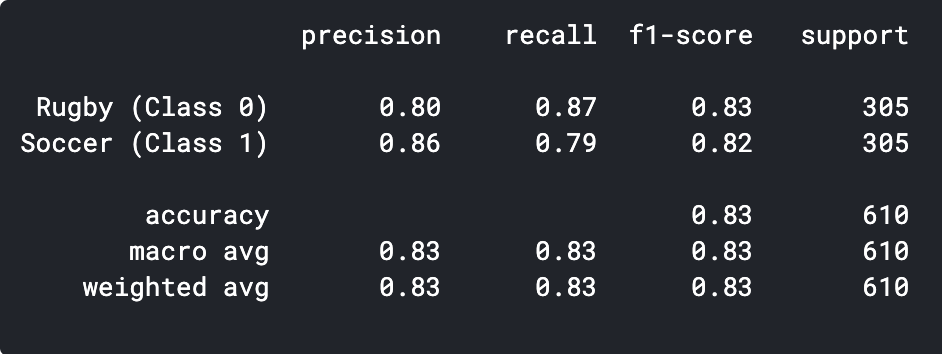As we can see our simple CNN model was able to achieve an accuracy of 83%. With some hyperparameter tuning, we might be able to achieve 2-3% accuracy.

We can also visualize some of the incorrectly predicted images and see where our classifier is going wrong.

## The Art of Transfer Learning

Let’s see what transfer learning is first. Transfer learning is a machine learning technique where a model trained on one task is re-purposed on a second related task. Another crucial application of transfer learning is when the dataset is small, by using a pre-trained model on similar images we can easily achieve high performance. Since our problem statement is a good fit for transfer learning lets see how we can go about implementing a pre-trained model and what accuracy we are able to achieve.

#### Step 1:- Import the model

We will create a base model from the MobileNetV2 model. This is pre-trained on the ImageNet dataset, a large dataset consisting of 1.4M images and 1000 classes. This base of knowledge will help us classify Rugby and Soccer from our specific dataset.

By specifying the include_top=False argument, you load a network that doesn’t include the classification layers at the top.

`base_model = tf.keras.applications.MobileNetV2(input_shape = (224, 224, 3), include_top = False, weights = "imagenet")`

It is important to freeze our base before we compile and train the model. Freezing will prevent the weights in our base model from being updated during training.

`base_model.trainable = False`

Next, we define our model using our base_model followed by a GlobalAveragePooling function to convert the features into a single vector per image. We add a dropout of 0.2 and the final dense layer with 2 neurons and softmax activation.

```model = tf.keras.Sequential([base_model,
tf.keras.layers.GlobalAveragePooling2D(),
tf.keras.layers.Dropout(0.2),
tf.keras.layers.Dense(2, activation="softmax")
])```

Next, let’s compile the model and start training it.

```base_learning_rate = 0.00001
loss=tf.keras.losses.BinaryCrossentropy(from_logits=True),
metrics=['accuracy'])

history = model.fit(x_train,y_train,epochs = 500 , validation_data = (x_val, y_val))```

#### Step 2:- Evaluating the result

```acc = history.history['accuracy']
val_acc = history.history['val_accuracy']
loss = history.history['loss']
val_loss = history.history['val_loss']
epochs_range = range(500)

plt.figure(figsize=(15, 15))
plt.subplot(2, 2, 1)
plt.plot(epochs_range, acc, label='Training Accuracy')
plt.plot(epochs_range, val_acc, label='Validation Accuracy')
plt.legend(loc='lower right')
plt.title('Training and Validation Accuracy')

plt.subplot(2, 2, 2)
plt.plot(epochs_range, loss, label='Training Loss')
plt.plot(epochs_range, val_loss, label='Validation Loss')
plt.legend(loc='upper right')
plt.title('Training and Validation Loss')
plt.show()```

Let’s see what the curve looks like:-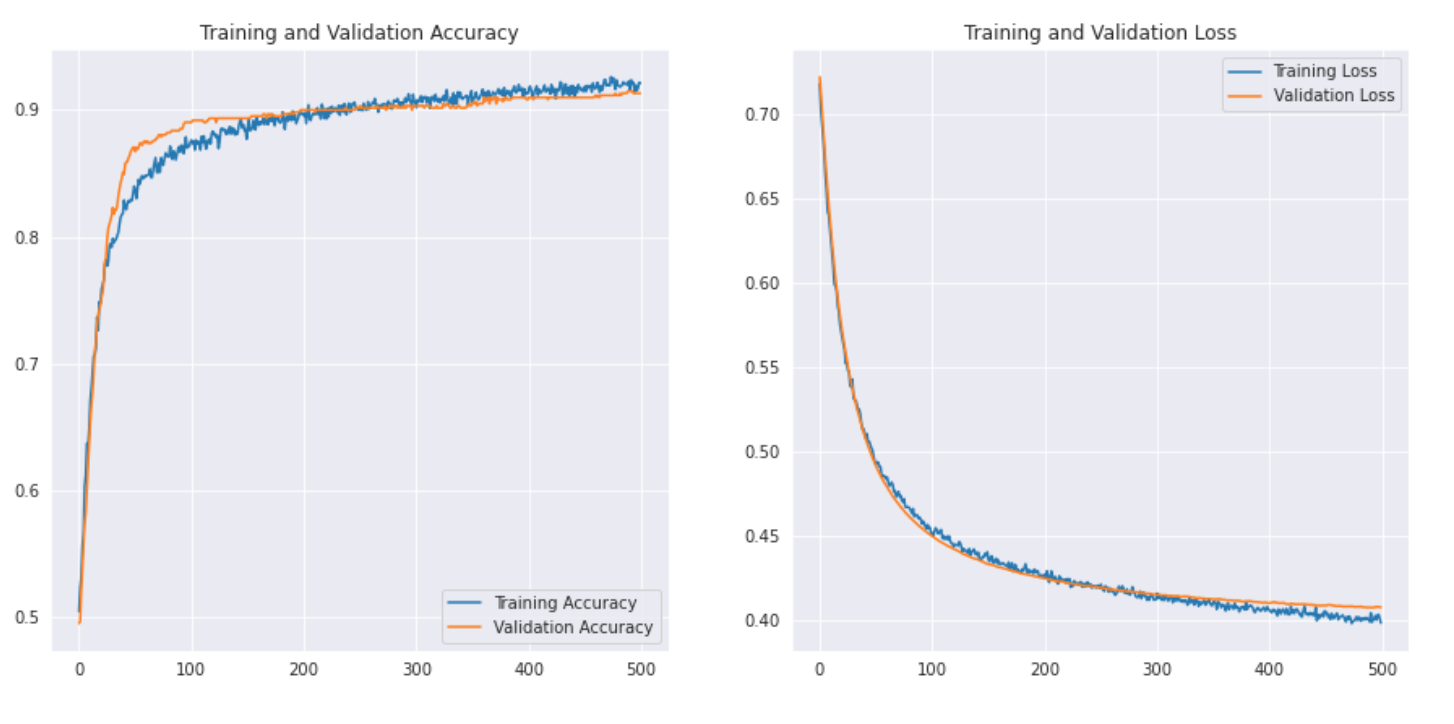Let’s also print the classification report to get more detailed results.

```predictions = model.predict_classes(x_val)
predictions = predictions.reshape(1,-1)

print(classification_report(y_val, predictions, target_names = ['Rugby (Class 0)','Soccer (Class 1)']))```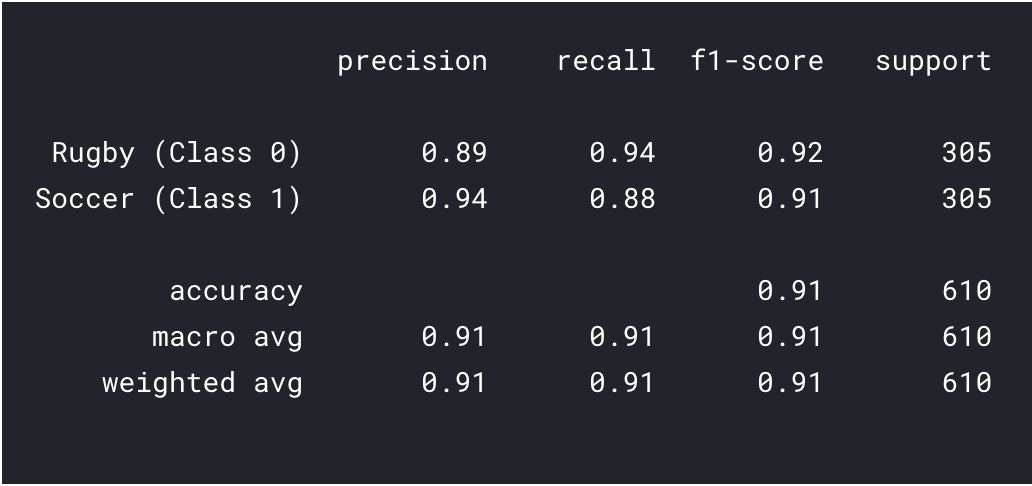As we can see with transfer learning we were able to get a much better result. Both the Rugby and Soccer precision are higher than our CNN model and also the overall accuracy reached 91% which is really good for such a small dataset. With a bit of hyperparameter tuning and changing parameters, we might be able to achieve a little better performance too!

## What’s Next?

This is just the starting point in the field of computer vision. In fact, try and improve your base CNN models to match or beat the benchmark performance.

• You can learn from the architectures of VGG16, etc for some clues on hyperparameter tuning.
• You can use the same ImageDataGenerator to augment your images and increase the size of the dataset.
• Also, you can try implementing newer and better architectures like DenseNet and XceptionNet.
• You can also move onto other computer vision tasks such as object detection and segmentation which u will realize later can also be reduced to image classification.

## End Notes

Congratulations you have learned how to make a dataset of your own and create a CNN model or perform Transfer learning to solving a problem. We learned a great deal in this article, from learning to find image data to create a simple CNN model that was able to achieve reasonable performance. We also learned the application of transfer learning to further improve our performance.

That is not the end, we saw that our models were misclassifying a lot of images which means that is still room for improvement. We could begin with finding more data or even implementing better and latest architectures that might be better at identifying the features.###### Tanishq Gautam

I am a 22 year old Computer Vision Enthusiast. I intend to improve and contribute to current technology and open new avenues in the computing industry.

## 18 thoughts on "Create your Own Image Classification Model using Python and Keras"###### Friedbert says:October 18, 2020 at 11:17 pm
Hallo Tanishg, I have no experience with the sources of the pictures. Can you give me a hint how I can download the pictures. Friedbert Reply###### Dai Software says:October 19, 2020 at 10:51 am
Wonderful Blog. multi vendor ecommerce website Reply###### Tanishq Gautam says:October 19, 2020 at 11:07 am###### Tanishq Gautam says:October 19, 2020 at 11:17 am###### Gopal krishna debnath says:November 24, 2020 at 9:22 pm
Excellent.lots of learning. Very important. Reply###### Tanishq Gautam says:December 03, 2020 at 8:24 pm###### Hun says:December 04, 2020 at 5:19 am
Hi, what would I need to change in the code if I have 5 (airplane, car, bus, cat, dog) classes instead of the 2 (rugby, soccer) you used in the first set of code? Running this: history = model.fit(x_train,y_train,epochs = 5 , validation_data = (x_val, y_val)) Gives me this error: Received a label value of 4 which is outside the valid range of [0, 2). Label values: 3 0 4 1 4 0 3 3 3 2 0 3 2 1 3 4 0 1 0 0 2 1 3 2 0 4 3 4 4 2 4 1 [[node sparse_categorical_crossentropy/SparseSoftmaxCrossEntropyWithLogits/SparseSoftmaxCrossEntropyWithLogits (defined at :1) ]] [Op:__inference_train_function_926] Function call stack: train_function I assume I need to change the model somehow, but I don't know what to change. Please assist. Thanks! Reply###### Tanishq Gautam says:January 04, 2021 at 10:34 am
Hi, Firstly, you will need to change the labels in the get_data() function when loading the data to include all 5 classes. Secondly, during the model creation, the final dense layer neurons represent the number of classes. For your case you will have to change the final dense layer neurons to 5 instead of 2. Reply###### Marley says:January 23, 2021 at 2:32 pm
Hello , plz could help me to find code of semantic segmentation on python Reply###### Ram Singh Chauhan says:January 23, 2021 at 8:16 pm
Running into this error while doing the transfer learning.. ValueError: A target array with shape (128, 1) was passed for an output of shape (None, 2) while using as loss `binary_crossentropy`. This loss expects targets to have the same shape as the output. Reply###### Tanishq Gautam says:January 25, 2021 at 6:38 pm
Hi, You have defined 1 neuron in the final layer, but 2 are expected as it is a binary classification problem with softmax activation. Reply###### Tanishq Gautam says:January 27, 2021 at 11:39 am
Hi, You can find a tutorial on semantic segmentation here:- https://www.analyticsvidhya.com/blog/2019/02/tutorial-semantic-segmentation-google-deeplab/ Reply###### Mohanad Khalid says:February 08, 2021 at 5:46 pm
Hi I am beginner, can you please give the exact code for downloading the data and how did you exactly connected the project code to the dataset location.. Thanks.. Reply###### Tanishq Gautam says:February 08, 2021 at 5:53 pm